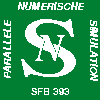Research Group Computational Methods in Systems and Control Theory

Diese Seite wird nicht mehr aktualisiert. Bitte besuchen Sie unsere neue Webpräsenz.# Parallel numerical solution of optimal control problems with instationary diffusion-convection-reaction equations

### partial project A15 of SFB393 Parallele Numerische Simulation für Physik und Kontinuumsmechanik

worked on by: Prof. Dr. Peter Benner, Dipl. Math. Jens SaakThis project considers boundary value problems with linear partial differetial equations and boundary control.

previous work:
In his diplom thesis Jens Saak worked on the controlled cooling of steel profiles during production process looking at railprofiles as an example. It was taken into concern that different areas of the surface of the material should be cooled differently intense.

The starting ponit from the view of an engineer was the fact that an equally distributed temperatureprofile on some cross-section of the profile before the last rolling established by selective cooling leads to better mechanical material characteristics of the endproduct than using conventional rolling without selective cooling.The rising mathematical problem is that of optimal control using boundary control for a (linearised) heat-equation. The variational formulation leads to a linear quadratic optimal control problem in classical formulation which in contrary to classical problems is formulated in an infinite dimensional hilbert-space. This problem due to Gibson 1979 can be solved by a feedback approach.

The Numerical implementation uses fem-semidiscretisation in space. This lead to a lqr-problem for an ordinary differential equation. This equation has Avery large (>1000) dimension which leads to additional difficulties for the numerical treatment.